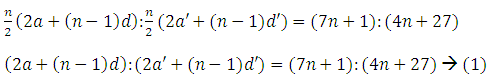Click to Chat

1800-1023-196

+91-120-4616500

CART 0

• 0

MY CART (5)

Use Coupon: CART20 and get 20% off on all online Study Material

ITEM
DETAILS
MRP
DISCOUNT
FINAL PRICE
Total Price: Rs.

There are no items in this cart.
Continue Shopping
`        The ratio of the sum of n terms of twm AP`s is (7n+1): (4n+1) find the ratio of their mth terms.`
one year ago

```							Given ratio of sum of n terms of two AP’s = (7n+1):(4n+27)Let’s consider the ratio these two AP’s mth terms as am : a’m →(2)Recall the nth term of AP formula, an = a + (n – 1)dHence equation (2) becomes,am : a’m = a + (m – 1)d : a’ + (m – 1)d’On multiplying by 2, we getam : a’m = [2a + 2(m – 1)d] : [2a’ + 2(m – 1)d’]= [2a + {(2m – 1) – 1}d] : [2a’ + {(2m – 1) – 1}d’]= S2m – 1 : S’2m – 1 = [7(2m – 1) + 1] : [4(2m – 1) +27] [from (1)]= [14m – 7 +1] : [8m – 4 + 27]= [14m – 6] : [8m + 23]Thus the ratio of mth terms of two AP’s is [14m – 6] : [8m + 23].
```
one year ago
Think You Can Provide A Better Answer ?

## Other Related Questions on 10 grade maths

View all Questions »### Course Features

• 728 Video Lectures
• Revision Notes
• Previous Year Papers
• Mind Map
• Study Planner
• NCERT Solutions
• Discussion Forum
• Test paper with Video Solution### Course Features

• 731 Video Lectures
• Revision Notes
• Test paper with Video Solution
• Mind Map
• Study Planner
• NCERT Solutions
• Discussion Forum
• Previous Year Exam Questions